How Cheenta works to ensure student success?
Explore the Back-Story

# NMTC 2017 Stage II - KAPREKAR (Class 7, 8) - Problems and Solutions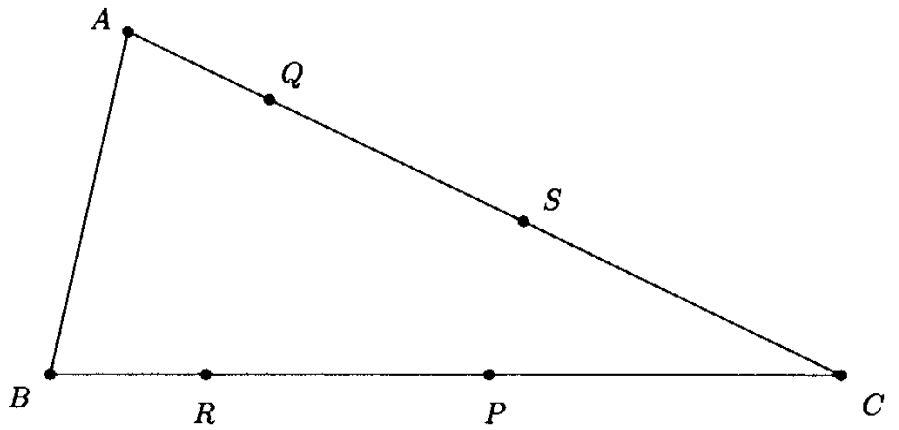###### Problem 1

(a) Find all three digit numbers in which any two adjacent digits differ by 3.
(b) There are 5 cards. Five positive integers (may be different or equal) are written on these cards, one on each card. Abhiram finds the sum of the numbers on every pair of cards. He obtains only three different totals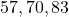. Find the largest integer written on a card.

###### Problem 2

(a)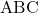is a triangle in which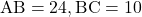and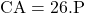is a point inside the triangle. Perpendiculars are drawn to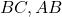and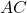. Length of these perpendiculars respectively are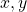and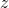. Find the numerical value of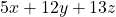.
(b) If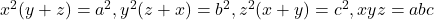prove that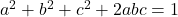###### Problem 3

IfFind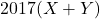###### Problem 4

The sum of the ages of a man and his wife is six times the sum of the ages of their children. Two years ago the sum of their ages was ten times the sum of the ages of their children. Six years hence the sum of their ages will be three times the sum of the ages of their children. How many children do they have?

###### Problem 5

(a)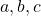are three natural numbers such that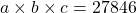. If, find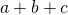.
(b)is a regular octagon with side length equal to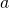. Find the area of the trapezium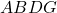.

###### Problem 6

(a) Ifare positive real number such that no two of them are equal, show thatis always positive
(b) In the figure below,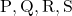are point on the sides of the trianglesuch that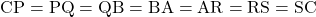###### Problem 1

(a) Find all three digit numbers in which any two adjacent digits differ by 3.
(b) There are 5 cards. Five positive integers (may be different or equal) are written on these cards, one on each card. Abhiram finds the sum of the numbers on every pair of cards. He obtains only three different totals. Find the largest integer written on a card.

###### Problem 2

(a)is a triangle in whichandis a point inside the triangle. Perpendiculars are drawn toand. Length of these perpendiculars respectively areand. Find the numerical value of.
(b) Ifprove that###### Problem 3

IfFind###### Problem 4

The sum of the ages of a man and his wife is six times the sum of the ages of their children. Two years ago the sum of their ages was ten times the sum of the ages of their children. Six years hence the sum of their ages will be three times the sum of the ages of their children. How many children do they have?

###### Problem 5

(a)are three natural numbers such that. If, find.
(b)is a regular octagon with side length equal to. Find the area of the trapezium.

###### Problem 6

(a) Ifare positive real number such that no two of them are equal, show thatis always positive
(b) In the figure below,are point on the sides of the trianglesuch thatThis site uses Akismet to reduce spam. Learn how your comment data is processed.

### 2 comments on “NMTC 2017 Stage II - KAPREKAR (Class 7, 8) - Problems and Solutions”

1.S. S. Maitra says:

Nice to develop a skill in mathematical thinking.

2.S. S. Maitra says:

Nice problems for young genii.

### Knowledge Partner